• BK-V: Measurements of B-mode Polarization at Degree Angular Scales and 150 GHz by Keck Array
The Keck Array and BICEP2 Collaborations, ApJ 811, 126, 2015
Individual receiver of the Keck Array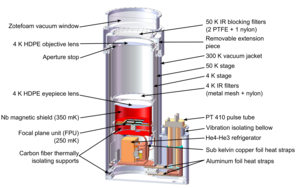Figure 1: Individual receiver of the Keck Array. Each receiver is cryogenic, with a pulse tube refrigerator cooling the optics to 4 K and a three-stage sorption refrigerator cooling the focal plane to 270 mK. The Keck Array consists of five identical receivers on a single telescope mount at the South Pole. PDF / PNG
Observing time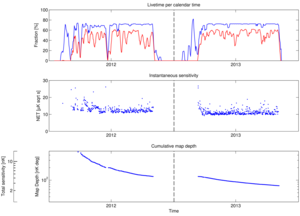Figure 2: The Keck Array 2012–2013 150 GHz data set. The top panel shows the fraction of calendar time the telescopes were observing. The red line represents the fraction of time preserved after all data selection cuts are implemented. The middle panel shows the instantaneous sensitivity of the full Keck Array. The bottom panel shows the cumulative map depth as calculated over each phase (10 hr of data). PDF / PNG
Keck Array T, Q, U maps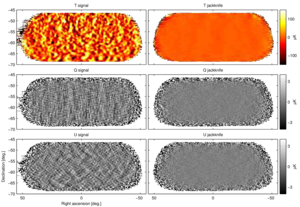Figure 3: Keck Array T, Q, U maps. The left column shows the basic signal maps with 0.25° pixelization as output by the reduction pipeline. The right column shows difference (jackknife) maps made with the first halves of the 2012 and 2013 seasons and the second halves. No additional filtering other than that imposed by the instrument beam (FWHM 0.5°) has been done. Note that the structure seen in the Q and U signal maps is as expected for an E-mode dominated sky. PDF / PNG
Power spectrum jackknife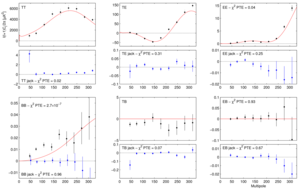Figure 4: Keck Array power spectrum results for signal (black points) and early/late season jackknife (blue points). The solid red curves show the lensed-ΛCDM theory expectations. The error bars are the standard deviations of the lensed-ΛCDM+noise simulations and hence contain no sample variance on any additional signal component. The probability to exceed (PTE) the observed value of a simple χ2 statistic for the 9 band powers is given (as evaluated against the simulations). Note that the band powers of the auto spectra of the simulations are approximately χ2 distributed, with the lowest ℓ-bin only containing ∼9 effective degrees of freedom. This increases the probability of an outlier point in comparison to a Gaussian distribution. The observed distribution for the cross spectra are more symmetric than the χ2 distributions but have similarly increased tails. This is fully reflected in the quoted PTE value. Also note the very different y-axis scales for the jackknife spectra (other than BB). See the text for additional discussion of the BB spectrum. (Note that the calibration procedure uses EB to set the overall polarization angle so TB and EB as plotted above cannot be used to measure astrophysical polarization rotation.) PDF / PNG
E-mode and B-mode maps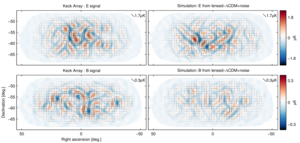Figure 5: Left: Keck Array apodized E-mode and B-mode maps filtered to 50<ℓ<120. Right: The equivalent maps for the first of the lensed-ΛCDM+noise simulations. The color scale displays the E-mode scalar and B-mode pseudoscalar patterns while the lines display the equivalent magnitude and orientation of linear polarization. Note that the E-mode and B-mode maps use different color/length scales. PDF / PNG
Jackknife PTE distributions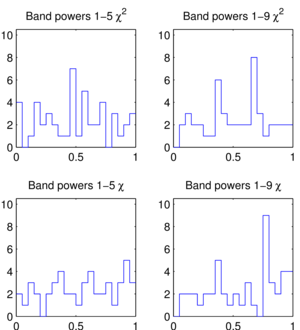Figure 6: Distributions of the jackknife χ2 and χ PTE values over the tests and spectra given in Table 4. PDF / PNG
BB spectra from T-only input simulations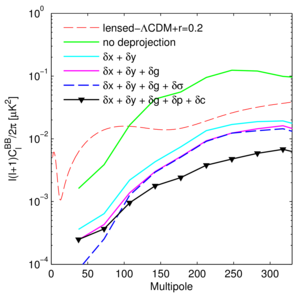Figure 7: BB spectra from T-only input simulations using the measured per channel beam shapes compared to the lensed-ΛCDM+r=0.2 spectrum. From top to bottom the curves are (i) no deprojection, (ii) deprojection of differential pointing only (δ x + δ y), (iii) deprojection of differential pointing and differential gain of the detector pairs (δ x + δ y + δ g), (iv) adding deprojection of differential beam width (δ x + δ y + δ g + δσ), and (v) differential pointing, differential gain, and differential ellipticity (δ x + δ y + δ g + δ p + δ c). This last curve represents an upper limit only to the residual contamination. PDF / PNG
Comparison of BICEP2 and Keck Array BB spectra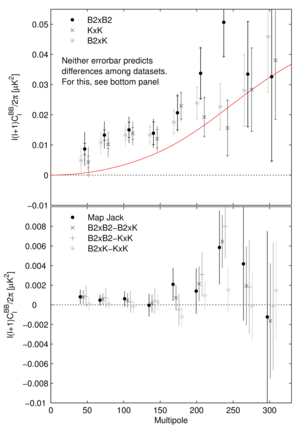Figure 8: Upper: The Keck Array BB auto spectrum, the BICEP2 auto spectrum, and the cross spectrum taken between the two. The inner error bars are the standard deviation of the lensed-ΛCDM+noise simulations, while the outer error bars also contain excess power at low-ℓ. (For clarity the Keck Array and cross spectrum points are offset horizontally.) Lower: Four compatibility tests between the B modes measured by BICEP2 and Keck Array. The “map jack” takes the difference of the Q and U maps, divides by a factor of two, and calculates the BB spectrum. The other three sets of points are the differences of the spectra shown in the upper panel divided by a factor of four. In each case the error bars are the standard deviation of the pairwise differences of signal+noise simulations which share common input skies. Comparison of any one of these sets of points with null is an appropriate test of the compatibility of the experiments—see text for details. All tests show good consistency between BICEP2 and Keck Array, particularly in the lowest five band powers. PDF / PNG
BB power spectrum of combined BICEP2 and Keck Array maps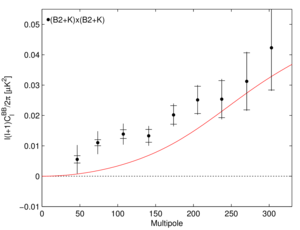Figure 9: The BB power spectrum of combined BICEP2 and Keck Array maps. The inner error bars are the standard deviation of the lensed-ΛCDM+noise simulations, while the outer error bars also contain excess power at low-ℓ. PDF / PNG

### History

• 2015-09-29: Updated figures and captions to as-published version.
• 2015-02-02: Posted BK-V: Measurements of B-mode Polarization at Degree Angular Scales and 150 GHz by Keck Array figures.